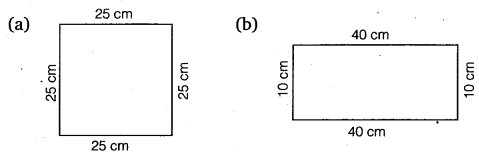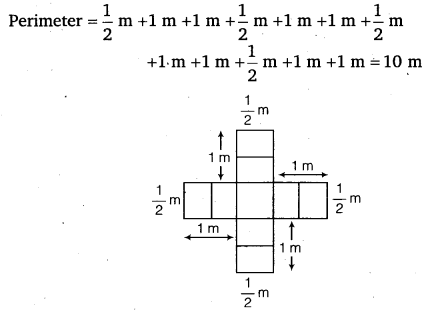# Class 6 Maths NCERT Solutions for Chapter 10 Mensuration Ex – 10.1

## Mensuration

Question 1.
Find the perimeter of each of the following figures:Solution:
(a) Perimeter = the sum of the lengths of sides
= 5 cm +1 cm +2 cm + 4 cm = 12cm
(b) Perimeter = the sum of the lengths of sides
= 40 cm +35 cm + 23 cm +35 cm = 133 cm
(c) Perimeter = 4x the length of one side
= 4 x 15 cm = 60 cm
(d) Perimeter = 5 x the length of one side
4 = 5 x 4 cm = 20 cm
(e) Perimeter = the sum of the lengths of sides
= 4 cm + 0.5 cm + 2.5 cm + 2.5 cm + 0.5 cm + 4 cm + 1 cm = 15 cm
(f) Perimeter = the sum of the lengths of sides
= 4 cm + 3 cm + 2 cm + 3 cm + 1 cm + 4 cm + 3 cm + 2 cm + 3 cm + 1 cm + 4 cm + 3 cm + 2 cm +3 cm +1 cm + 4 cm + 3 cm + 2 cm + 3 cm + 1 cm = 52 cm

Question 2.
The lid of a rectangular box of sides 40 cm by 10 cm is sealed all round with tape. What is the length of the tape required?

Solution:
Length of the tape required
= Perimeter of the lid of a rectangular box = 2 x ( length + breadth)
= 2 x (40 cm +10 cm)
= 2 x 50 cm = 100 cm or 1 m

Question 3.
A table-top measures 2 m 25 cm by 1 m 50 cm. What is the perimeter of the table-top?

Solution:
Perimeter of the table-top
= 2 x (length + breadth)
= 2 x (2 m 25 cm +1 m 50 cm)
= 2 x (3 m 75 cm)
= 2 x 3.75 m = 7.50 m

Question 4.
What is the length of the wooden strip required to frame a photograph of length and breadth 32 cm and 21 cm, respectively?

Solution:
The length of the wooden strip required to frame a photograph is the perimeter of the photograph.
Perimeter of the photograph = 2 x (length + breadth)
= 2 x (32 cm +21 cm)
= 2 x 53 cm = 106 cm
∴ The length of the wooden strip required is 106 cm.

Question 5.
A rectangular piece of land measures 0.7 km by 0.5 km. Each side is to be fenced with 4 rows of wires. What is the length of the wire needed?

Solution:
We have to cover 4 times the perimeter of the land measuring 0.7 km by 0.5 km.
∴ Total length of wire required is 4 times its perimeter.
Perimeter of the land = 2 x (length + breadth)
= 2x (0.7 km+0.5 km)
= 2 x 1.2 km = 2.4 km
∴ Total length of wire required = 4 x 2.4 km = 9.6 km

Question 6.
Find the perimeter of each of the following shapes:

(a) A triangle of sides 3 cm, 4 cm and 5 cm.
(b) An equilateral triangle of side 9 cm.
(c) An isosceles triangle with equal sides 8 cm each and third side 6 cm.

Solution:
(a) Perimeter = the sum of the sides
= 3 cm + 4 cm + 5cm = 12 cm
(b) Perimeter = 3 x the length of one side
= 3 x 9 cm = 27 cm
(c) Perimeter = the sum of the lengths of the sides
= 8 cm + 8 cm + 6 cm = 22 cm

Question 7.
Find the perimeter of a triangle with sides measuring 10 cm, 14 cm and 15 cm.

Solution:
Perimeter of the triangle = the sum of the lengths of its sides
= 10 cm + 14 cm + 15 cm = 39 cm

Question 8.
Find the perimeter of a regular hexagon with each side measuring 8 m.

Solution:
A regular hexagon has 6 sides, so its perimeter
= 6 x length of its one side = 6 x 8 m
= 48 m

Question 9.
Find the side of the square whose perimeter is 20 m.

Solution:
Perimeter = 20 m
A square has 4 equal sides, so we can divide the perimeter by 4 to get the length of one side.
One side of the square = 20 m + 4 = 5m

Question 10.
The perimeter of a regular pentagon is 100 cm. How long is its each side?

Solution:
Perimeter = 100 cm
A regular pentagon has 5 equal sides, so we can divide the perimeter by
5 to get the length of one side
∴ length of one side = 100 cm + 5 = 20 cm

Question 11.
A piece of string is 30 cm long. What will be the length of each side if the string is used to forms

(a) a square?
(b) an equilateral triangle?
(c) a regular hexagon?

Solution:
(a) Perimeter = Length of the string = 30 cm
A square has 4 equal sides, so we can divide the perimeter by 4 to get the length of one side.
One side of the square = 30 cm + 4 = 7.5 cm

(b)
Perimeter = Length of the string = 30 cm
An equilateral triangle has 3 equal sides, so we can divide the perimeter by 3 to get the length of one side.
∴ One side of an equilateral triangle = 30 cm ÷ 3 = 10 cm
(c) Perimeter = Length of the string = 30 cm
A regular hexagon has 6 equal sides, so we can divide the perimeter by
6 to get the length of one side.
One side of a regular hexagon = 30 cm + 6 = 5 cm

Question 12.
Two sides of a triangle are 12 cm and 14 cm. The perimeter of the triangle is 36 cm. What is its third side?

Solution:
Let ABC be the given triangle such that AB =12 cm, BC = 14 cm and its perimeter = 36 cm.
i.e., AB + BC + CA = 36 cm
or 12 cm + 14 cm + CA = 36 cm
or 26 cm + CA = 36 cm
or CA =36 cm – 26 cm = 10 cm
∴ The third side of triangle is 10 cm.

Question 13.
Find the cost of fencing a square park offside 250 mat the rate of ? 20 per metre.

Solution:
Side of the square park = 250 m
∴ Perimeter of the square park
= 4 x side
= 4 x 250 m = 1000 m
∴ Cost of fencing = ₹ (1000 x 20)
= ₹ 20000

Question 14.
Find the cost of fencing a rectangular park of length 175 m and breadth 125 m at the rate of? 12 per metre.

Solution:
Length of the rectangular park = 175 m Breadth of the rectangular park 125 m .-. Perimeter of the park = 2 x (length + breadth)
= 2 x (175m + 125m)
= 2 x 300 m = 600 m
∴ Cost of fencing = ₹ (600 x 12) = ₹ 7200

Question 15.
Sweety runs around a square park of side 75 m. Bulbul runs around a rectangular park with length 60 m and breadth 45 m. Who covers less distance?

Solution:
The distance each girl covers in one round is the same as the perimeter of the respective field. Therefore, the distance that Sweety covers in one round
= 4 x side
= 4 x 75 m = 300 m
Also, the distance that Bulbul covers in one round
= 2 x (length + breadth)
= 2 x (60 m + 45 m)
= 2 x 105 m = 210 m
This shows that Bulbul covers less distance than Sweety.

Question 16.
What is the perimeter of each of the following figures? What do you infer from the answer?Solution:
(a) Perimeter = 4 x side
= 4 x 25 cm
= 100 cm
(b) Perimeter = 2 x (length + breadth)
= 2 x (40 cm +10 cm)
= 2 x 50 cm = 100 cm
(c) Perimeter = 2 x (length + breadth)
2 x (30 cm +20 cm)
= 2 x 50 cm = 100 cm
(d) Perimeter = 30 cm +30 cm +40 cm = 100 cm
Thus, we observe that the perimeter of each figure is 100 cm i.e., they have equal perimeters.

Question 17.
Avneet buys 9 square paving slabs, each with a side of 1/2 m. He lays them in the form of a square.

(a)
What is the perimeter of his arrangement [Fig. (i)]?
(b) Shari does not like his arrangement. She gets him to lay them out like a cross. What is the perimeter of her arrangement [Fig. (ii)]?
(c) Which has greater perimeter?
(d) Avneet wonders if there is a way of getting an even greater perimeter. Can you find a way of doing this? (The paving slabs must meet along complete edges i.e. they cannot be broken.)

Solution:
(a) In case of Avneet’s arrangement:(b) In case of Shari’s arrangement:(c) Clearly, perimeter in case of Shari is greater.
(d) Yes, there is a way shown in the figure in which we get a greater perimeterPerimeter = 2 x (9 + 1) units = 2 x 10 units = 20 units